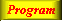Lecture 3 - Page 23 : 42
 Functional Programming in SchemeName binding, Recursion, Iteration, and Continuations * Name binding constructs The let name binding expression The equivalent meaning of let Examples with let name binding The let* name binding construct An example with let* The letrec namebinding construct LAML time functions * Conditional expressions Conditional expressions Examples with if Example with cond: leap-year? Example with cond: american-time Example with cond: as-string * Recursion and iteration Recursion List processing Tree processing (1) Tree processing (2) Recursion versus iteration Example of recursion: number-interval Examples of recursion: string-merge Examples with recursion: string-of-char-list? Exercises * Example of recursion: Hilbert Curves Hilbert Curves Building Hilbert Curves of order 1 Building Hilbert Curves of order 2 Building Hilbert Curves of order 3 Building Hilbert Curves of order 4 A program making Hilbert Curves * Continuations Introduction and motivation The catch and throw idea A catch and throw example The intuition behind continuations Being more precise The capturing of continuations Capturing, storing, and applying continuations Use of continuations for escaping purposes Practical example: Length of an improper list Practical example: Searching a binary tree
 Examples with recursion: string-of-char-list?
 The function string-of-char-list? is a predicate (a boolean function) that finds out if a string is formed exclusively by characters from a particular alphabet.
 ```(define (string-of-char-list? str char-list) (string-of-char-list-1? str char-list 0 (string-length str))) (define (string-of-char-list-1? str char-list i lgt) (if (= i lgt) #t (and (memv (string-ref str i) char-list) (string-of-char-list-1? str char-list (+ i 1) lgt))))```

The function string-of-char-list? which relies on the tail recursive function string-of-char-list-1?. The function string-of-char-list-1? iterates through the characters in str, via the controlling parameters i and lst.Applications of string-of-char-list?.

The function blank-string? determines if a string is formed entirely of white space characters. The function numeric-string? is a predicate that returns true if the string consists exclusively of decimal digits. This is, for instance, useful to check the form input of dates and time in some server-based web applications. The version of numeric-string? in the lib/general.scm of LAML is slightly more general than the version shown above (it allows + or - signs as well, depending on an optional parameter).